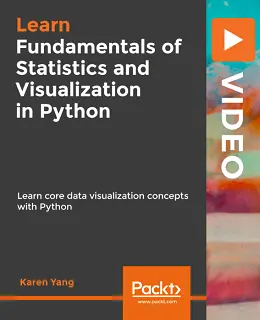# Fundamentals of Statistics and Visualization in Python [Video]### Fundamentals of Statistics and Visualization in Python [Video]

##### English | MP4 | AVC 1920×1080 | AAC 48KHz 2ch | 3h 15m | 752 MB eLearning | Skill level: All Levels

Fundamentals of Statistics and Visualization in Python [Video]: Learn to display your data using Python’s visualization tools

Statistics and visualization in Python can be applied to a wide variety of areas; having these skills is crucial for data scientists. In this course, we explore several core statistical concepts to utilize data; construct confidence intervals in Python and assess the results; discover correlations; and update your beliefs using Bayesian Inference.

In this tutorial, you will discover how to use the Statsmodels, Matplotlib, pandas, and Seaborn Python libraries for statistical data visualization. Follow along with author – Dr. Karen Yang, a seasoned data scientist and data engineer – to explore, learn, and strengthen your skills in fundamental statistics and visualization. This course utilizes the Jupyter Notebook environment to execute tasks.

By the end of this learning journey, you’ll have developed a solid understanding of fundamental statistics and visualization concepts and will be confident enough to apply them to your data analysis projects.

• Basic concepts in statistics and data visualization
• Use Python data visualization tools to perform data visualization
• Apply probability to statistics with the use of Bayesian Inference, a powerful alternative to classical statistics
• Calculate and build confidence intervals in Python
• Run basic regressions focused on linear and multilinear data
• Run hypothesis tests and perform Bayesian inference for effective analysis and visualization
• Apply probability to statistics by updating beliefs

Please note that prior knowledge of Python programming and some familiarity with pandas and NumPy are needed in order to get the best out of this course.